# The ROBUSTREG Procedure

### M Estimation

This example shows how you can use the ROBUSTREG procedure to do M estimation, which is a commonly used method for outlier detection and robust regression when contamination is mainly in the response direction.

The following data set, Stack, is the well-known stack loss data set presented by Brownlee (1965). The data describe the operation of a plant for the oxidation of ammonia to nitric acid and consist of 21 four-dimensional observations. The explanatory variables for the response stack loss (y) are the rate of operation (x1), the cooling water inlet temperature (x2), and the acid concentration (x3).

data Stack;
input  x1 x2 x3 y exp \$ @@;
datalines;
80  27   89  42   e1   80  27   88  37   e2
75  25   90  37   e3   62  24   87  28   e4
62  22   87  18   e5   62  23   87  18   e6
62  24   93  19   e7   62  24   93  20   e8
58  23   87  15   e9   58  18   80  14  e10
58  18   89  14  e11   58  17   88  13  e12
58  18   82  11  e13   58  19   93  12  e14
50  18   89   8  e15   50  18   86   7  e16
50  19   72   8  e17   50  19   79   8  e18
50  20   80   9  e19   56  20   82  15  e20
70  20   91  15  e21
;


The following PROC ROBUSTREG statements analyze the data:

proc robustreg data=stack;
model y = x1 x2 x3 / diagnostics leverage;
id    exp;
test  x3;
run;


By default, the ROBUSTREG procedure uses the bisquare weight function to do M estimation, and it uses the median method to estimate the scale parameter. The MODEL statement specifies the covariate effects. The DIAGNOSTICS option requests a table for outlier diagnostics, and the LEVERAGE option adds leverage-point diagnostic results to this table for continuous covariate effects. The ID statement specifies that the variable exp be used to identify each observation (experiment) in this table. If the ID statement is omitted, the observation number is used to identify the observations. The TEST statement requests a test of significance for the covariate effects that are specified. The results of this analysis are displayed in Figures Figure 98.1 through Figure 98.3.

Figure 98.1 displays the model-fitting information and summary statistics for the response variable and the continuous covariates. The Q1, Median, and Q3 columns provide the lower quantile, median, and upper quantile, respectively. The MAD column provides a robust estimate of the univariate scale, which is computed as the standardized median absolute deviation (MAD). For more information about the standardized MAD, see Huber (1981, p. 108). The Mean and Standard Deviation columns provide the usual mean and standard deviation. A large difference between the standard deviation and the MAD for a variable indicates some extreme values for that variable. In the stack loss data, the stack loss (response y) has the biggest difference between the standard deviation and the MAD.

Figure 98.1: Model-Fitting Information and Summary Statistics

The ROBUSTREG Procedure

Model Information
Data Set WORK.STACK
Dependent Variable y
Number of Independent Variables 3
Number of Observations 21
Method M Estimation

Summary Statistics
Variable Q1 Median Q3 Mean Standard
Deviation
MAD
x1 53.0000 58.0000 62.0000 60.4286 9.1683 5.9304
x2 18.0000 20.0000 24.0000 21.0952 3.1608 2.9652
x3 82.0000 87.0000 89.5000 86.2857 5.3586 4.4478
y 10.0000 15.0000 19.5000 17.5238 10.1716 5.9304

Figure 98.2 displays the table of robust parameter estimates, standard errors, and confidence limits. The Scale row provides a point estimate of the scale parameter in the linear regression model, which is obtained by the median method. For more information about scale estimation methods, see the section M Estimation. For the stack loss data, M estimation yields the fitted linear model: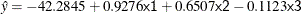Figure 98.2: Model Parameter Estimates

Parameter Estimates
Parameter DF Estimate Standard
Error
95% Confidence Limits Chi-Square Pr > ChiSq
Intercept 1 -42.2854 9.5045 -60.9138 -23.6569 19.79 <.0001
x1 1 0.9276 0.1077 0.7164 1.1387 74.11 <.0001
x2 1 0.6507 0.2940 0.0744 1.2270 4.90 0.0269
x3 1 -0.1123 0.1249 -0.3571 0.1324 0.81 0.3683
Scale 1 2.2819

Figure 98.3 displays outlier and leverage-point diagnostics. Standardized robust residuals are computed based on the estimated parameters. Both the Mahalanobis distance and the robust MCD distance are displayed. Outliers and leverage points, identified by asterisks, are defined by the standardized robust residuals and robust MCD distances that exceed the corresponding cutoff values displayed in the diagnostics summary. Observations 4 and 21 are outliers because their standardized robust residuals exceed the cutoff value in absolute value. The procedure detects four observations that have high leverage values. Leverage points (points with high leverage values) that have smaller standardized robust residuals than the cutoff value in absolute value are called good leverage points; others are called bad leverage points. Observation 21 is a bad leverage point.

Figure 98.3: Diagnostics

Diagnostics
Obs exp Mahalanobis Distance Robust MCD Distance Leverage Standardized
Robust Residual
Outlier
1 e1 2.2536 5.5284 * 1.0995
2 e2 2.3247 5.6374 * -1.1409
3 e3 1.5937 4.1972 * 1.5604
4 e4 1.2719 1.5887   3.0381 *
21 e21 2.1768 3.6573 * -4.5733 *

Two particularly useful plots for revealing outliers and leverage points are a scatter plot of the standardized robust residuals against the robust distances (RD plot) and a scatter plot of the robust distances against the classical Mahalanobis distances (DD plot).

For the stack loss data, the following statements produce the RD plot in Figure 98.4 and the DD plot in Figure 98.5. The histogram and the normal quantile-quantile plots (shown in Figure 98.6 and Figure 98.7, respectively) for the standardized robust residuals are also created using the HISTOGRAM and QQPLOT suboptions of the PLOTS= option.

ods graphics on;

proc robustreg data=stack plots=(rdplot ddplot histogram qqplot);
model y = x1 x2 x3;
run;

ods graphics off;


These plots are helpful in identifying outliers in addition to good and bad high-leverage points.

These plots are requested when ODS Graphics is enabled by specifying the PLOTS= option in the PROC ROBUSTREG statement. For general information about ODS Graphics, see Chapter 21: Statistical Graphics Using ODS. For specific information about the graphics available in the ROBUSTREG procedure, see the section ODS Graphics.

Figure 98.4: RD Plot for Stack Loss Data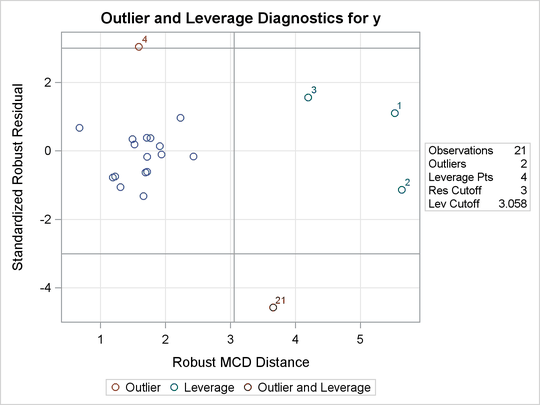Figure 98.5: DD Plot for Stack Loss Data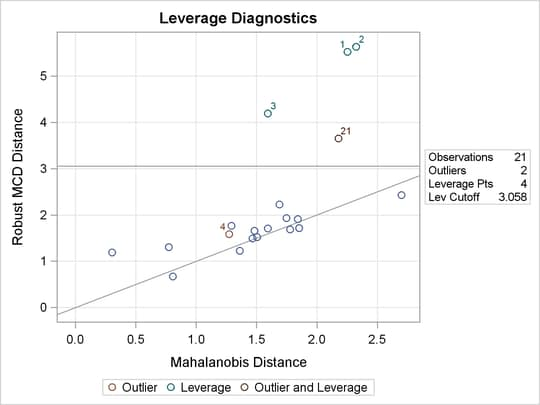Figure 98.6: Histogram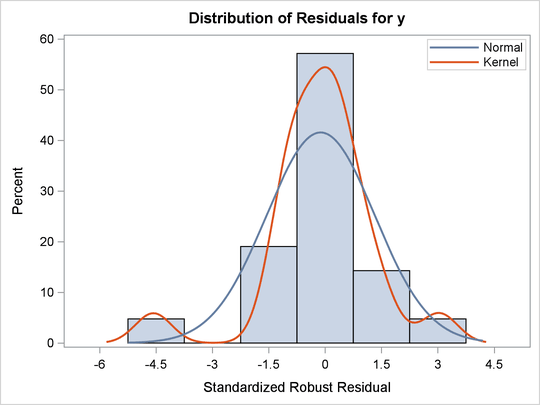Figure 98.7: Q-Q Plot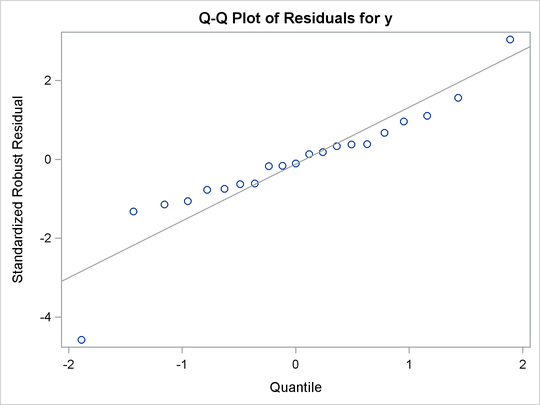Figure 98.8 displays robust versions of goodness-of-fit statistics for the model. You can use the robust information criteria, AICR and BICR, for model selection and comparison. For both AICR and BICR, the lower the value, the more desirable the model.

Figure 98.8: Goodness-of-Fit Statistics

Goodness-of-Fit
Statistic Value
R-Square 0.6659
AICR 29.5231
BICR 36.3361
Deviance 125.7905

Figure 98.9 displays the test results that are requested by the TEST statement. The ROBUSTREG procedure conducts two robust linear tests, the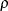test and the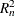test. For information about how the ROBUSTREG procedure computes test statistics and the correction factor lambda, see the section Linear Tests. Because of the large p-values for both tests, you can conclude that the effect x3 is not significant at the 5% level.

Figure 98.9: Test of Significance

Robust Linear Test
Test Test Statistic Lambda DF Chi-Square Pr > ChiSq
Rho 0.9378 0.7977 1 1.18 0.2782
Rn2 0.8092   1 0.81 0.3683

For the bisquare weight function, the default tuning constant, c = 4.685, is chosen to yield a 95% asymptotic efficiency of the M estimates with the Gaussian distribution. For more information, see the section M Estimation. The smaller the constant c, the lower the asymptotic efficiency but the sharper the M estimate as an outlier detector. For the stack loss data set, you could consider using a sharper outlier detector.

The following PROC ROBUSTREG step uses a smaller constant, c = 3.5. This tuning constant corresponds to an efficiency close to 85%. For the relationship between the tuning constant and asymptotic efficiency of M estimates, see Chen and Yin (2002).

proc robustreg method=m(wf=bisquare(c=3.5)) data=stack;
model y = x1 x2 x3 / diagnostics leverage;
id    exp;
test  x3;
run;


Figure 98.10 displays the table of robust parameter estimates, standard errors, and confidence limits when PROC ROBUSTREG uses the constant c = 3.5.

The refitted linear model is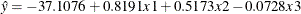Figure 98.10: Model Parameter Estimates

The ROBUSTREG Procedure

Parameter Estimates
Parameter DF Estimate Standard
Error
95% Confidence Limits Chi-Square Pr > ChiSq
Intercept 1 -37.1076 5.4731 -47.8346 -26.3805 45.97 <.0001
x1 1 0.8191 0.0620 0.6975 0.9407 174.28 <.0001
x2 1 0.5173 0.1693 0.1855 0.8492 9.33 0.0022
x3 1 -0.0728 0.0719 -0.2138 0.0681 1.03 0.3111
Scale 1 1.4265

Figure 98.11 displays outlier and leverage-point diagnostics when the constant c = 3.5 is used. Besides observations 4 and 21, observations 1 and 3 are also detected as outliers.

Figure 98.11: Diagnostics

Diagnostics
Obs exp Mahalanobis Distance Robust MCD Distance Leverage Standardized
Robust Residual
Outlier
1 e1 2.2536 5.5284 * 4.2719 *
2 e2 2.3247 5.6374 * 0.7158
3 e3 1.5937 4.1972 * 4.4142 *
4 e4 1.2719 1.5887   5.7792 *
21 e21 2.1768 3.6573 * -6.2727 *